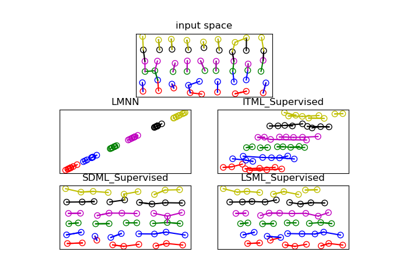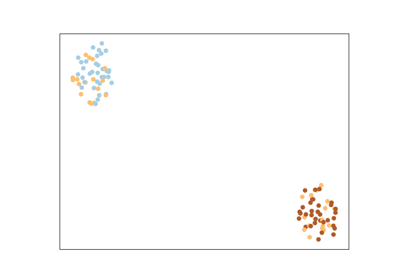# metric_learn.ITML_Supervised¶

class metric_learn.ITML_Supervised(gamma=1.0, max_iter=1000, convergence_threshold=0.001, num_constraints=None, prior='identity', verbose=False, preprocessor=None, random_state=None)[source]

Supervised version of Information Theoretic Metric Learning (ITML)

ITML_Supervised creates pairs of similar sample by taking same class samples, and pairs of dissimilar samples by taking different class samples. It then passes these pairs to ITML for training.

Parameters: gammafloat, optional (default=1.0)Value for slack variables max_iterint, optional (default=1000)Maximum number of iterations of the optimization procedure. convergence_thresholdfloat, optional (default=1e-3)Tolerance of the optimization procedure. num_constraintsint, optional (default=None)Number of constraints to generate. If None, default to 20 * num_classes**2. priorstring or numpy array, optional (default=’identity’)Initialization of the Mahalanobis matrix. Possible options are ‘identity’, ‘covariance’, ‘random’, and a numpy array of shape (n_features, n_features). For ITML, the prior should be strictly positive definite (PD). ‘identity’An identity matrix of shape (n_features, n_features). ‘covariance’The inverse covariance matrix. ‘random’The prior will be a random SPD matrix of shape (n_features, n_features), generated using sklearn.datasets.make_spd_matrix. numpy arrayA positive definite (PD) matrix of shape (n_features, n_features), that will be used as such to set the prior. verbosebool, optional (default=False)If True, prints information while learning preprocessorarray-like, shape=(n_samples, n_features) or callableThe preprocessor to call to get tuples from indices. If array-like, tuples will be formed like this: X[indices]. random_stateint or numpy.RandomState or None, optional (default=None)A pseudo random number generator object or a seed for it if int. If prior='random', random_state is used to set the prior. In any case, random_state is also used to randomly sample constraints from labels.

metric_learn.ITML
The original weakly-supervised algorithm
Supervised versions of weakly-supervised algorithms
The section of the project documentation that describes the supervised version of weakly supervised estimators.

Examples

>>> from metric_learn import ITML_Supervised
>>> X = iris_data['data']
>>> Y = iris_data['target']
>>> itml = ITML_Supervised(num_constraints=200)
>>> itml.fit(X, Y)

Attributes: bounds_numpy.ndarray, shape=(2,)Bounds on similarity, aside slack variables, s.t. d(a, b) < bounds_ for all given pairs of similar points a and b, and d(c, d) > bounds_ for all given pairs of dissimilar points c and d, with d the learned distance. If not provided at initialization, bounds_ and bounds_ are set at train time to the 5th and 95th percentile of the pairwise distances among all points in the training data X. n_iter_intThe number of iterations the solver has run. components_numpy.ndarray, shape=(n_features, n_features)The linear transformation L deduced from the learned Mahalanobis metric (See function components_from_metric.)

Methods

 fit(X, y[, bounds]) Create constraints from labels and learn the ITML model. fit_transform(X[, y]) Fit to data, then transform it. get_mahalanobis_matrix() Returns a copy of the Mahalanobis matrix learned by the metric learner. get_metric() Returns a function that takes as input two 1D arrays and outputs the learned metric score on these two points. get_params([deep]) Get parameters for this estimator. score_pairs(pairs) Returns the learned Mahalanobis distance between pairs. set_params(**params) Set the parameters of this estimator. transform(X) Embeds data points in the learned linear embedding space.
__init__(gamma=1.0, max_iter=1000, convergence_threshold=0.001, num_constraints=None, prior='identity', verbose=False, preprocessor=None, random_state=None)[source]

Initialize self. See help(type(self)) for accurate signature.

fit(X, y, bounds=None)[source]

Create constraints from labels and learn the ITML model.

Parameters: X(n x d) matrixInput data, where each row corresponds to a single instance. y(n) array-likeData labels. boundsarray-like of two numbersBounds on similarity, aside slack variables, s.t. d(a, b) < bounds_ for all given pairs of similar points a and b, and d(c, d) > bounds_ for all given pairs of dissimilar points c and d, with d the learned distance. If not provided at initialization, bounds_ and bounds_ will be set to the 5th and 95th percentile of the pairwise distances among all points in the training data X.
fit_transform(X, y=None, **fit_params)

Fit to data, then transform it.

Fits transformer to X and y with optional parameters fit_params and returns a transformed version of X.

Parameters: X{array-like, sparse matrix, dataframe} of shape (n_samples, n_features) yndarray of shape (n_samples,), default=NoneTarget values. **fit_paramsdictAdditional fit parameters. X_newndarray array of shape (n_samples, n_features_new)Transformed array.
get_mahalanobis_matrix()

Returns a copy of the Mahalanobis matrix learned by the metric learner.

Returns: Mnumpy.ndarray, shape=(n_features, n_features)The copy of the learned Mahalanobis matrix.
get_metric()

Returns a function that takes as input two 1D arrays and outputs the learned metric score on these two points.

This function will be independent from the metric learner that learned it (it will not be modified if the initial metric learner is modified), and it can be directly plugged into the metric argument of scikit-learn’s estimators.

Returns: metric_funfunctionThe function described above.

score_pairs
a method that returns the metric score between several pairs of points. Unlike get_metric, this is a method of the metric learner and therefore can change if the metric learner changes. Besides, it can use the metric learner’s preprocessor, and works on concatenated arrays.

Examples

>>> from metric_learn import NCA
>>> from sklearn.datasets import make_classification
>>> from sklearn.neighbors import KNeighborsClassifier
>>> nca = NCA()
>>> X, y = make_classification()
>>> nca.fit(X, y)
>>> knn = KNeighborsClassifier(metric=nca.get_metric())
>>> knn.fit(X, y)
KNeighborsClassifier(algorithm='auto', leaf_size=30,
metric=<function MahalanobisMixin.get_metric.<locals>.metric_fun
at 0x...>,
metric_params=None, n_jobs=None, n_neighbors=5, p=2,
weights='uniform')

get_params(deep=True)

Get parameters for this estimator.

Parameters: deepbool, default=TrueIf True, will return the parameters for this estimator and contained subobjects that are estimators. paramsmapping of string to anyParameter names mapped to their values.
score_pairs(pairs)

Returns the learned Mahalanobis distance between pairs.

This distance is defined as: $$d_M(x, x') = \sqrt{(x-x')^T M (x-x')}$$ where M is the learned Mahalanobis matrix, for every pair of points x and x'. This corresponds to the euclidean distance between embeddings of the points in a new space, obtained through a linear transformation. Indeed, we have also: $$d_M(x, x') = \sqrt{(x_e - x_e')^T (x_e- x_e')}$$, with $$x_e = L x$$ (See MahalanobisMixin).

Parameters: pairsarray-like, shape=(n_pairs, 2, n_features) or (n_pairs, 2)3D Array of pairs to score, with each row corresponding to two points, for 2D array of indices of pairs if the metric learner uses a preprocessor. scoresnumpy.ndarray of shape=(n_pairs,)The learned Mahalanobis distance for every pair.

get_metric
a method that returns a function to compute the metric between two points. The difference with score_pairs is that it works on two 1D arrays and cannot use a preprocessor. Besides, the returned function is independent of the metric learner and hence is not modified if the metric learner is.
Mahalanobis Distances
The section of the project documentation that describes Mahalanobis Distances.
set_params(**params)

Set the parameters of this estimator.

The method works on simple estimators as well as on nested objects (such as pipelines). The latter have parameters of the form <component>__<parameter> so that it’s possible to update each component of a nested object.

Parameters: **paramsdictEstimator parameters. selfobjectEstimator instance.
transform(X)

Embeds data points in the learned linear embedding space.

Transforms samples in X into X_embedded, samples inside a new embedding space such that: X_embedded = X.dot(L.T), where L is the learned linear transformation (See MahalanobisMixin).

Parameters: Xnumpy.ndarray, shape=(n_samples, n_features)The data points to embed. X_embeddednumpy.ndarray, shape=(n_samples, n_components)The embedded data points.

## Examples using metric_learn.ITML_Supervised¶